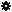﻿ Object Model: PolygonMesh.GetPolygonIndexArray

PolygonMesh.GetPolygonIndexArray

## PolygonMesh.GetPolygonIndexArrayv5.0

### Description

Returns the polygon indices on which point locators are defined.

Notice that this information is part of polygon mesh point locator's definition, and depends on the topology only (won't change if the geometry is deformed).

The position within the polygon can be queried with methods PolygonMesh.GetTriangleVertexIndexArray, PolygonMesh.GetTriangleNodeIndexArray and PolygonMesh.GetTriangleWeightArray.

### C# Syntax

 ```Object PolygonMesh.GetPolygonIndexArray( PointLocatorData in_pPtLocators, Object in_pPointLocatorsIndices ); ```

### Scripting Syntax

 ```oArray = PolygonMesh.GetPolygonIndexArray( PointLocators, [Indices] ); ```

### Return Value

1-dimensional Array

### Parameters

Parameter Type Description
PointLocators PointLocatorData Point locators to be described.
Indices 1-dimensional Array Indices of the point locators to be described (all if not specified).

### Examples

#### JScript Example

 ```// // This example topologically describes the point locators resulting // from the shrink-wrapping of a cube onto a mesh sphere. // NewScene(); var root = Application.ActiveSceneRoot; var CubePositionArray = root.AddGeometry("Cube", "MeshSurface").ActivePrimitive.Geometry.Points.PositionArray; var MeshSphereGeom = root.AddGeometry("Sphere", "MeshSurface").ActivePrimitive.Geometry; var CubeOnSpherePointLocators = MeshSphereGeom.GetClosestLocations(CubePositionArray); var PtLocIndices = new Array(1); for(i = 0; i < CubeOnSpherePointLocators.Count; i++) { PtLocIndices = i; var PtLocPolygon = MeshSphereGeom.GetPolygonIndexArray( CubeOnSpherePointLocators, PtLocIndices).toArray(); var PtLocSubTriNodes = MeshSphereGeom.GetTriangleNodeIndexArray( CubeOnSpherePointLocators, PtLocIndices).toArray(); var PtLocSubTriVertices = MeshSphereGeom.GetTriangleVertexIndexArray( CubeOnSpherePointLocators, PtLocIndices).toArray(); var PtLocBaryCoords = MeshSphereGeom.GetTriangleWeightArray( CubeOnSpherePointLocators, PtLocIndices).toArray(); Application.LogMessage("Point locator " + i + " is on polygon " + PtLocPolygon + ","); Application.LogMessage("on a subtriangle described by nodes (" + PtLocSubTriNodes + ", " + PtLocSubTriNodes + ", " + PtLocSubTriNodes + ")" + " or by vertices (" + PtLocSubTriVertices + ", " + PtLocSubTriVertices + ", " + PtLocSubTriVertices + ")"); Application.LogMessage("and having barycentric coordinates (" + PtLocBaryCoords + ", " + PtLocBaryCoords + ", " + PtLocBaryCoords + ")"); Application.LogMessage(""); } // Expected results: //INFO : Point locator 0 is on polygon 58, //INFO : on a subtriangle described by nodes (217, 219, 220) or by vertices (52, 4, 53) //INFO : and having barycentric coordinates (0.6173165440559387, 0, 0.3826834559440613) //INFO : //INFO : Point locator 1 is on polygon 42, //INFO : on a subtriangle described by nodes (157, 159, 160) or by vertices (38, 46, 39) //INFO : and having barycentric coordinates (0.6173165440559387, 0, 0.3826834559440613) //INFO : //INFO : Point locator 2 is on polygon 61, //INFO : on a subtriangle described by nodes (229, 231, 232) or by vertices (55, 7, 56) //INFO : and having barycentric coordinates (0.3826834261417389, 0, 0.6173166036605835) //etc. ```